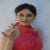# Hans B Pacejka

Updated on
Covid-19
 Name  Hans PacejkaBooks  Tyre and vehicle dynamics

Hans Bastiaan Pacejka (born 1934) is an expert in vehicle system dynamics and particularly in tire dynamics, fields in which his works are now standard references. He is Professor emeritus at Delft University of Technology in Delft, Netherlands.

## The Pacejka "Magic Formula" tire models

Pacejka has developed a series of tire design models over the last 20 years. They were named the 'magic formula' because there is no particular physical basis for the structure of the equations chosen, but they fit a wide variety of tire constructions and operating conditions. Each tire is characterized by 10-20 coefficients for each important force that it can produce at the contact patch, typically lateral and longitudinal force, and self-aligning torque, as a best fit between experimental data and the model. These coefficients are then used to generate equations showing how much force is generated for a given vertical load on the tire, camber angle and slip angle.

The Pacejka tire models are widely used in professional vehicle dynamics simulations, and racing car games, as they are reasonably accurate, easy to program, and solve quickly. A problem with Pacejka's model is that when implemented into computer code, it doesn't work for low speeds (from around the pit-entry speed), because a velocity term in the denominator makes the formula diverge. An alternative to Pacejka tire models are brush tire models, which can be analytically derived, although empirical curve fitting is still required for good correlation, and they tend to be less accurate than the MF models.

The general form of the magic formula is: R ( k ) = d s i n { c a r c t a n [ b ( 1 e ) k + e a r c t a n ( b k ) ] } where b, c, d and e represent fitting constants and R is a force or moment resulting from a slip parameter k.

Hans B. Pacejka Wikipedia

Topics